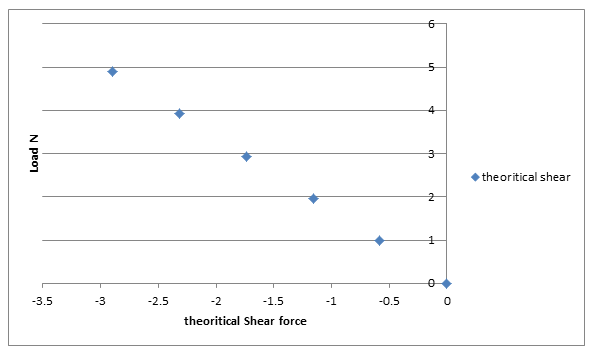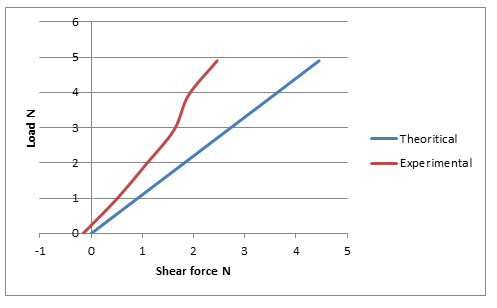# Shear Force in a Beam Lab ReportAim
Aim of this experiment is to study the effect of force magnitude on shear forces in a beam
Theory
Shear force
In beam designing shear force is one which is perpendicular to the longitudinal x axis of beam. Shear force is more important that axial force in beam designing.

Introduction to experiment
This experiment is about understanding the effect of change in force magnitude on shear stresses produce in beam due to that force. To perform this experiment structural hardware ‘STR3 shear force in a beam’ is used. Following the figure of structural hardware ‘STR3 shear force in a beam’

Figure 5 Shear stress apparatusAccording to the image shown above, apparatus consist of a beam which is being supported at two points called pivot point of beam. The apparatus has a mechanism which is used to apply force on the beam and it is this mechanism which also prevents the overloading on beam which can collapse the beam. During this experiment a series of forces with increasing magnitude will be applied on beam and for each beam shear stress value will be calculated.  Free body diagram of this apparatus has been shown below.

Figure 6 Shear stress apparatus free body diagramshear stress free body diagram

As this experiment is about calculating or observing the shear for on beam due to the externally applied forces on beam so following the formula for this beam needed to calculate the shear force produce in beam by the external force.

Shear force = (W(a))/l

Here
W is a the applied load on beam
a is the distance between the pivot point and point of force application.
l is the total length of the beam

Procedure

• Following are the steps involve in this hear force calculation of beam. It is very important to following the sequence of these steps as this experiment involve electronic system which is integrated with software for shear force application and calculation.
• First step is to setup the apparatus which involve starting the computer software according to the guide provided and setting it to virtual experiment mode
• Second step is to select the variable hanger load which is available in the property selection box
• Third step involves the placement of load at the required distance from one pivot point of beam
• Data generated as the result of force application is recorded by the software automatically
• Third step is repeated for the different loads and for each load software record data automatically in its memory
• Graphs were prepared using the data obtain from the experiment

Results

Table 3 Shear stress calculations

 Mass g Load N Distance from left mm Experimental Shear at cut N Theoretical shear at cut N Theoretical reaction at left support N Theoretical reaction at right support N 0 0 400 -0.16 0.00 0.00 0.00 100 0.98 400 0.50 -0.58 0.40 0.58 200 1.96 400 1.07 -1.16 0.80 1.16 300 2.94 400 1.62 -1.74 1.20 1.74 400 3.92 400 1.90 -2.32 1.60 2.32 500 4.90 400 2.46 -2.90 2.01 2.90

Calculations
Following is the equation which can be used for the shear force calculation

Shear force = (W(a))/l

Here
W is a the applied load on beam

a is the distance between the pivot point and point of force application = 400

l is the total length of the beam = 440

For W = 0
Shear force = (W* a)/l=(0*400)/440=0 N

For W = 0.98
Shear force = (W* a)/l=(0.98*400)/440=0.8909 N

For W = 1.96
Shear force = (W* a)/l=(1.96*400)/440=1.7817 N

For W = 2.94
Shear force = (W* a)/l=(2.94*400)/440=2.6727 N

For W = 3.92
Shear force = (W* a)/l=(3.92*400)/440=3.5636 N

For W = 4.90
Shear force = (W* a)/l=(4.90*400)/440=4.45 N

Percentage error
Percentage Error= PE = (Experimental shear force-Theoretical shear force)/(Theoretical shear force) × 100%

PE =  (0.50-0.8909)/0.8909  × 100%

PE =-43.84 %

Table 4 Shear stress calculations

 Load (N) Theoretical Shear Force, Sc (N) Experimental Shear Force (N) % Error 0 0 -0.16 0.00 0.98 0.8909 0.50 -43.84 1.96 1.7817 1.07 -39.94 2.94 2.6727 1.62 -39.38 3.92 3.5636 1.90 -46.68 4.90 4.45 2.46 -44.71

Figure 7 Shear stress graphShear stresses in beam

Figure 8 Shear stress comparison graphshear stress in beam comparison

Discussion
The first is between load and the shear force with load is on y axis and shear force is on x axis. The graph trend show that the relationship between load and shear force is directly proportional mean that with increase in load there is an increase in shear force and with decrease in load there is a decrease in shear force.

Second graph also between load and shear force with load is on y axis and shear force is on x axis but this graph is actually comparison between the experimental shear force and theoretical shear force. This comparison shows that there is great difference between the experimental shear force and theoretical shear force. Actual difference is in experimental values as they don’t show the linear relationship between loads and shear force. This error is due to many reasons like personal error due to unexperienced worker or faulty apparatus.

Conclusion
Aim of this experiment was to study the effect of different force magnitude on shear forces produce in beam due to the force. Theoretical calculation of shear force show that there is a direct relation between load and shear force but the experimental shear force show some errors which can be due to personal error or faulty apparatus.